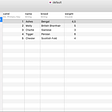# Learning to Code: Day 52 — Basic JavaScript Part 17

Hello everyone (and welcome to the new people who joined yesterday!), I hope you are all doing well, today we’re going to look a little into different types of random number generating, then we’ll move onto the parseInt() function used in JavaScript and a bit about Radix. Finally we’ll check out the Conditional Operator, also known as the Ternary Operator.

Thanks as always to FreeCodeCamp for the lessons.

## Generating Random Fractions

If we want to create random behaviour in JS, we can do so by creating random numbers. The Math.random() function generates a random decimal number between and including 0 up to but not including 1. Let’s take a look at that in it’s simplest form:

## Generating Random Whole Numbers

It’s one thing to generate random decimal numbers, but what if we wanted to generate random whole numbers instead?

We can do this by rounding a decimal number down to the nearest whole number, using the Math.floor() function.

Say we want to take a random decimal number created by the Math.random() function, multiply it by 20 and then round it down to a whole number by passing the total into the Math.floor() function, it would look like so:

Remember: Because Math.random() can never give us a whole number, when that decimal is multiplied by 20 it can never give us 20, so the final number will be somewhere between 0 and 19.

## Generating Random Whole Numbers within a Range

With everything we now know so far, we can apply a minimum and maximum range to the random number generator, which we define as arguments in the brackets of the function, in this example on line 1 as myMin and myMax:

Here we see the formula (provided by FreeCodeCamp) used to figure out how the range works, seen in line 3. Later on, when the user types in two values (seen on line 6),those two values will correspond to the two arguments myMin and myMax and be included in the formula itself. The result will give us a number within those two given numbers, inclusive. In this case, somewhere equal to or greater than 4 and equal to or less than 7.

## Using the parseInt() Function

The parseInt() function parses a string and returns an integer. If the first character in the string cannot be converted (if it’s a letter and not a number), it’ll return NaN (Not a Number).

So, it takes a string that is either a decimal number (like above) or beginning with 0 (e.g. 0000574 = 574) and rounds it down to the full whole number. The above example on line 5 will output “2”.

## Using the parseInt() Function with a Radix

According to Wikipedia:

In a positional numeral system, the radix or base is the number of unique digits, including the digit zero, used to represent numbers. For example, for the decimal/denary system the radix is ten, because it uses the ten digits from 0 through 9.

In the example below, we are inputting the binary number “10011”, and in the binary system, the base is 2 (because the number 0 and 1 are used). When we account for the radix as a secondary argument in the brackets of the parseInt() function, we are referring to it like this:

On line 3, the radix here, “2” is the base of the number in the string (seen as the first argument, str). This is telling the code that the string is in binary form. It’s worth noting that the radix can be an integer between 2 and 36, depending on the numerical system used.

Therefore, taking all this into account, the given binary example on line 6 will be output as 19 in the console.

## The Conditional/Ternary Operator

Now here’s a thing I’ve seen bouncing around JavaScript. I’ve always wondered what this symbol was. It’s the question mark (“?”) but looks like this in the following syntax:

`condition ? expression-if-true : expression-if-false;` FreeCodeCamp

Here we are saying “Here’s the condition, and if true then say.. otherwise say..” This symbol holds the same weight like that of an if/else statement but it’s alot cleaner and in one line.

Note: Check out the colon which (I think!) stands for “else”.

Let’s see this conditional operator in action on line 3:

## Using Multiple Conditional Operators

We can also chain multiple conditional operators together if we wanted, cause why not, right? Let’s see what an if/else statement converted over to chained conditional operators would look like:

This simplified would look like so:

It helps for me to read the code out loud in English to see how those conditional operators in the code are working. Let’s see.. “return a is strictly equal to b if (conditional operator) true then say a and b are equal, else(colon) say a is greater than b, if(conditional operator) true then say a is greater, otherwise(colon) say b is greater” if all above is false. Simple isn’t it? And don’t forget the semi-colon at the end!

Aaaand let’s end it on a good note there! Cheers everyone and I’ll catch you all next time! Hope you had fun, I know that the conditional operator was a big itch finally scratched!

See you!

--

--

--

## More from The Startup

Get smarter at building your thing. Follow to join The Startup’s +8 million monthly readers & +756K followers.

## Setting up a TLS server in NODE.js## Realm + Codable. Part 2.## Lodash vs ES6. Why Lodash still preferred.## Simplified ESLint API## Mentorship with Host Company## Hugh Burgess

Step by step, learning to code with FreeCodeCamp

## How to make Programming Fun?## Web Development’s Most Popular Programming Languages## 5 Tips for New Web Developers — From Other Web Developers